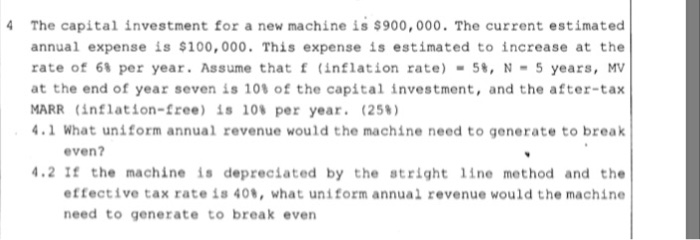Chat Now

# Engineering Economics 4 The capital investment for a new machine is \$900,000. The current estimated

### Engineering Economics 4 The capital investment for a new machine is \$900,000. The current estimated

Engineering Economics4 The capital investment for a new machine is \$900,000. The current estimated annual expense is \$100,000.This expense is estimated to increase at the rate of 6 per year. Assume that f (inflation rate) -5, N-5 years, Mv at the end of year seven is 10% of the capital investment, and the after-tax MARR (inflation-free) is 10 per year. (259) .1 What uniform annual revenue would the machine need to generate to break even? 4.2 If the machine is depreciated by the stright 1ine method and the effective tax rate is 408, what uniform annual revenue would the machine need to generate to break even

manish jayant 22-Apr-2020Get solution